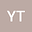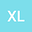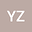Least-squares solutions of the generalized reduced biquaternion matrix equations
•••• Yong Tian,
• Xin LIU,
• Yang Zhang
Yong Tian
Macau University of Science and Technology

Corresponding Author:[email protected]

Author ProfileXin LIU
Macau University of Science and Technology
Author ProfileYang Zhang
Univ Manitoba
Author Profile## Abstract

In this paper, we introduce the definition of the generalized reduced biquaternions and propose a real representation of a generalized reduced biquaternion matrix. By using the matrix representation, we discuss the least-squares problems of the classic generalized reduced biquaternion matrix equation $AXC=B$. The least-squares solution to the above matrix equation is formulated by a least-squares real solution of its corresponding real matrix equation. Furthermore, two numerical examples are given to illustrate our results.